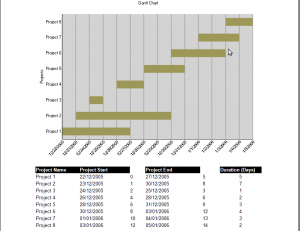﻿ How To: Create a Dynamic Gantt Chart# How To: Create a Dynamic Gantt Chart

One of the many chart types provided in ActiveReports is the Gantt Chart. The Gantt chart is a project management tool used to analyze the progress of individual project tasks. The chart shows a comparison of project task completion to the task schedule. In a Gantt chart the X and Y axes are reversed i.e the X axis is vertical and the Y axis is horizontal. In this blog post I would be discussing about creating a Gantt chart at run time. Before we actually start with the implementation, let us see how our final output will look like:For the ease of understanding the approach, we'll divide and discuss the implementation in two parts.

## Creating the DataSource

The first step is to set up a data source in order to provide data to the chart. Since we are creating the chart programmatically, we will use the ReportStart event to assign the datasource.

``````
private void rptProjects_ReportStart(object sender, System.EventArgs e)
{
String connString = @"Provider=Microsoft.Jet.OLEDB.4.0;Data Source=C:\\data.mdb;Persist Security Info=False";
// The sql statement for the DataTable (the datasource for the report and chart)
String rptSqlCmd = @"SELECT tblProjects.ProjectName, " +
"tblProjects.ProjectStart, " +
"tblProjects.ProjectEnd, " +
"DateDiff('d',[tblProjects]![ProjectStart],[tblProjects]![ProjectEnd]) AS Duration, " +
"DateDiff('d',[StartDate],[tblProjects]![ProjectStart]) AS StartValue, " +
"DateDiff('d',[StartDate],[tblProjects]![ProjectEnd]) AS EndValue " +
"FROM tblProjects;";
using (OleDbConnection conn = new OleDbConnection(connString))
{
conn.Open();
//The Gantt series takes two Y values: the start and end points as integers so
//we need to compute these from the StartDate and EndDate. To do the computation
//we need to subtract these dates from a starting date which is the
//minimum start date for the projects.
//Get the earliest project start date
OleDbCommand cmd1 = new OleDbCommand("SELECT Min([ProjectStart]) AS MinStartDate FROM tblProjects;", conn);
DateTime minStartDate = (DateTime)cmd1.ExecuteScalar();
// Get the latest project end date
OleDbCommand cmd2 = new OleDbCommand("SELECT Max([ProjectEnd]) AS MaxEndDate FROM tblProjects;", conn);
DateTime maxEndDate = (DateTime)cmd2.ExecuteScalar();
// Provide the minimum value as a parameter to the SQL statement.
// The SQL Statement has calculated fields StartValue and EndValue. These columns in the
// DataTable will be the ValueMemembersY of the series
OleDbCommand cmd = new OleDbCommand(rptSqlCmd, conn);
DataTable dtProjects = new DataTable("Projects");
da.Fill(dtProjects);
// Set the datatable as the datasource for the chart and report
this.DataSource = dtProjects;
SetChartControl(dtProjects, minStartDate, maxEndDate);
conn.Close();
}
}
``````

## Plotting Chart Data

When we are creating a chart, we also need to set various properties in order to achieve the desired results. Following code shows the required implementation:

``````private void SetChartControl(DataTable dt, DateTime minDate, DateTime maxDate)
{
// Chart Titles
this.chartControl1.Titles.Remove(this.chartControl1.Titles["footer"]);
// ValueMember
this.chartControl1.Series["Series1"].ValueMemberX = "ProjectName";
this.chartControl1.Series["Series1"].ValueMembersY = "StartValue, EndValue";
// AxisX properties
this.chartControl1.ChartAreas.Axes["AxisX"].Title = "Projects";
this.chartControl1.ChartAreas.Axes["AxisX"].TitleFont.Angle = -90;
// AxisY properties
GrapeCity.ActiveReports.Chart.AxisBase axisY = this.chartControl1.ChartAreas.Axes["AxisY"];
// Use the min and max dates to add labels to the y-axis
int labelCount = 0;
for (DateTime counter = minDate; counter <= maxDate; counter = counter.AddDays(1))
{
labelCount++;
}
axisY.Min = 0;
axisY.Max = labelCount - 1;
axisY.MajorTick.Step = 1;
axisY.MajorTick.Visible = true;
axisY.LabelsVisible = true;
axisY.MajorTick.Visible = true;
axisY.MajorTick.GridLine = new GrapeCity.ActiveReports.Chart.Graphics.Line(Color.Gray, 1, GrapeCity.ActiveReports.Chart.Graphics.LineStyle.Solid);
axisY.LabelFont.Angle = -45;
axisY.TitleFont.Angle = 0;
axisY.SmartLabels = false;
axisY.Title = "";
// Set the Chartcontrol Data Source
this.chartControl1.DataSource = dt;
}
``````